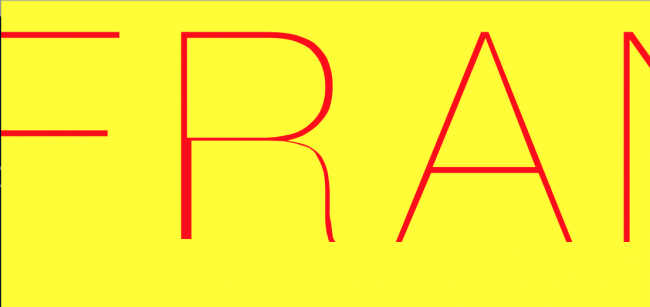#### Howdy, Stranger!

We are about to switch to a new forum software. Until then we have removed the registration on this forum.

# Why do SVG files load into Processing imperfectly?

edited May 2015

Hello.

SVG files seem to load into Processing with imperfections. In my case I am trying to load a piece of text created in Adobe Illustrator and saved out as an SVG into processing and from there save as PDF.

The code works fine, but the output of the image is not as I expected.

Here is a zoomed in view of the Illustrator file:Here is a zoomed in view of the PDF output:Any ideas on the reason for this?

Any help would be amazing!

Here is the code:

``````import processing.pdf.*;

//PShape template;
color[] colors = {#ff0000, #00ff00, #0000ff,#ffff00, #FFB9A0};
Curve bit = new Curve();
int cards = 50;

void setup(){
size(249,168);
println(PFont.list());
}

void draw(){
beginRecord(PDF, "data/something-####.pdf");
bit.bg();
bit.settings(40);//(weight)
bit.make(70,-15); //(gap, push)
//bit.rectangle(20);//(height)
bit.title();//(text, start, space)
endRecord();

if(frameCount == cards){
exit();
}
}

class Curve {

int c, e, gap, weight,push,h,space,start;
String name;
float r;
PFont f;

PShape template;

void settings(int weight_) {
weight = weight_;
e = color(colors[(int)random(colors.length)]);
noFill();
strokeWeight(weight);
stroke(e);
}

void make(int gap_, int push_) {
gap = gap_;
push = push_;
r=random(-100, 100);
curve(0, r, gap, height/2-push, width-gap, height/2-push, width, r);
}

void bg() {
c = color(colors[(int)random(colors.length)]);
background(c);
}

void rectangle(int h_){
h =  h_;
fill(e);
rect(0,0,width,h);
}

void title(){